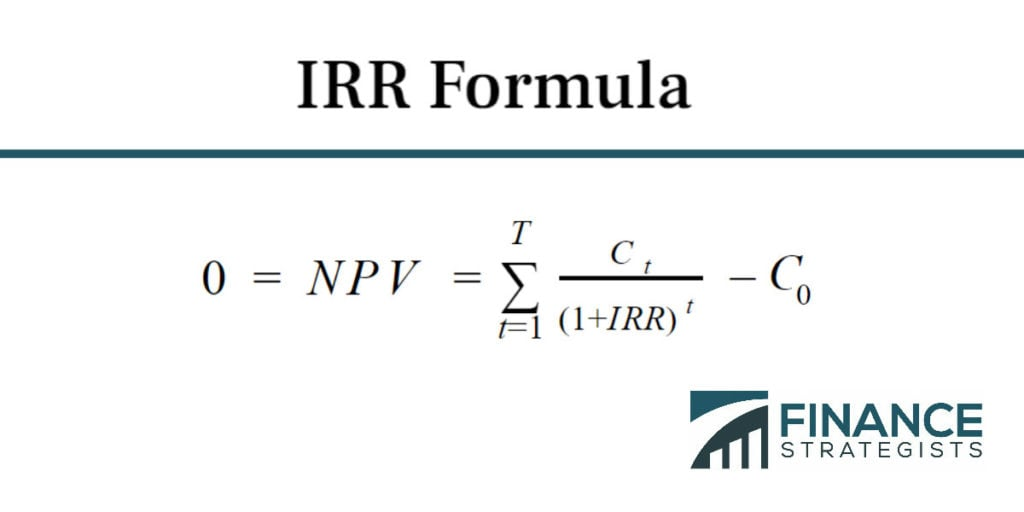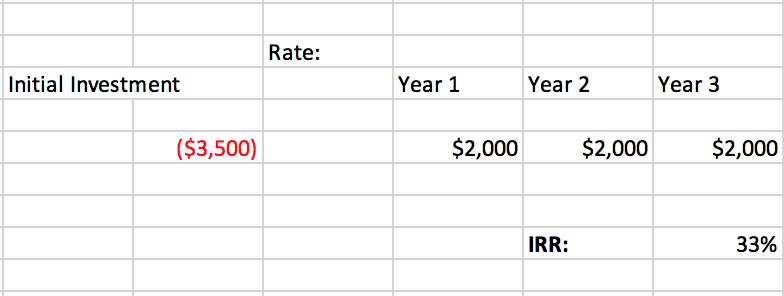# Internal Rate of Return (IRR) | IRR Formula### Written byTrue Tamplin, BSc, CEPF® | Reviewed by Editorial Team

Updated on December 23, 2022

## Calculate IRR

The internal rate of return is a financial metric that shows how profitable a project can be by determining the rate of return at which point the project would break even.

The formula for calculating IRR is very similar to that of calculating the net present value (NPV) because it essentially determines the discount rate--the rate of expected investment earnings--that would set the NPV equal to zero. The NPV represents the value of cash flows at a point in time in the past, present or (expected) future.

## Calculating IRR

The NPV is calculated by taking the total summation of the cash flow and then multiplying that by the dividend of net cash outflows divided by one plus the discount rate of return. It is a complex calculation usually done using computer software or advanced calculators.

## Internal Rate of Return Formula

The IRR calculation has the same structure as the NPV, except the NPV value is set to zero and the discount rate of return has to be solved for. Additionally, the most common form of the IRR formula has one subtract the initial investment value from the rest of the equation.

## IRR Formula## How to Calculate Internal Rate of Return

However, the IRR is usually calculated using a computer software tool like Microsoft Excel. To do so, one must first determine the value of the initial investment in a project and the yield of that investment over time. In order for the NPV to hit zero, the present value of future cash flows must equal the initial investment amount--anything above that is considered positive net profit value and inversely correlates with the discount rate, so a lower discount rate leads to a higher NPV.

## Calculation Necessity

All that is needed to calculate IRR in Excel is the initial investment amount and future cash yield per year. The present value of those future cash flows is not needed.

## IRR Example

For instance, if a company wanted to invest \$3,500 into a project that offered \$2,000 per year over three years, those numbers can be broken down into an excel spreadsheet for an IRR calculation like this:The initial investment is listed as a negative in order for Excel to know to calculate it against the expected annual return. Once the annual yield is listed, the IRR can be calculated by searching it in the functions menu in Excel and selecting the cells with the initial investment and annual yield as the input into the "Values" section.

## IRR Equation

The IRR equation allows an easy way for investors to see whether the discount rate and yield on an investment is worth pursuing without calculating the full NPV.

For instance, if the discount rate on the above hypothetical investment was 10%, a company would know even before calculating the full net profit value that the investment would be profitable because the proposed discount rate is far below the IRR. The company could also compare that IRR to that of other projects in order to quantify the benefit those projects--and the amount of money sunk in to them--could have for the overall company's finances.

Thus, while other financial metrics should be considered when weighing the pros and cons for leveraging finances into a project like this one, knowing how to find IRR can be an extremely useful skill.

## Internal Rate of Return (IRR) | IRR Formula FAQs

### What does IRR stand for?

IRR stands for internal rate of return.

### What is IRR?

The internal rate of return is a financial metric that shows how profitable a project can be by determining the rate of return at which point the project would break even.

### Why is IRR useful to investors?

The IRR equation allows an easy way for investors to see whether the discount rate and yield on an investment is worth pursuing without calculating the full NPV.

### What is the IRR formula?

The formula for IRR is complex, and so accountants usually use Excel. All that is needed to calculate IRR in Excel is the initial investment amount and future cash yield per year.

### How does a company use the IRR internally?

The company could compare the IRR of projects in order to quantify the benefit those projects – and the amount of money sunk in to them – could have for the overall company’s finances.